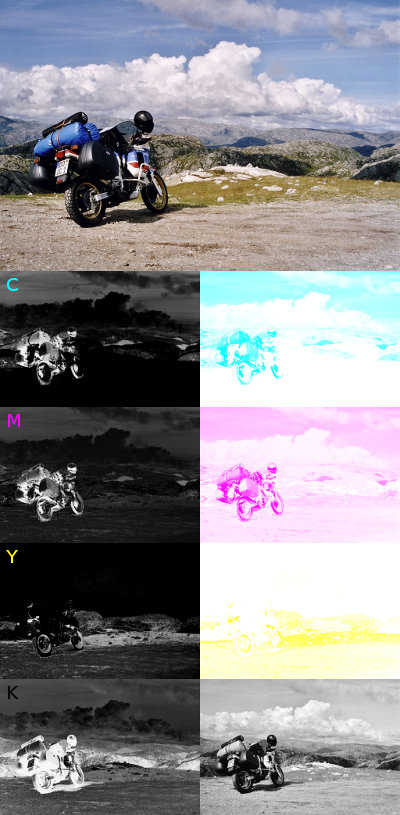# RGB to CMYKThe content of this page has not been vetted since shifting away from MediaWiki. If you’d like to help, check out the how to help guide!Natively, ImageJ supports RGB and HSL color spaces. There is no effort spent to support color management because the application is targeted at scientific image processing rather than image preparation for screen or print. For pure educational purposes, we show here how to do a trivial transformation between uncalibrated linear RGB to uncalibrated linear CMYK where, in RGB, the grey intensity is just (R+G+B)/3.

## Description

CMYK is a subtractive color space with a redundant gray channel to save color ink. The pure Cyan, Yellow and Magenta components are calculated by linearly combining the RGB components (in unsigned byte range [0…255]):

``````c = 1 - r / 255
m = 1 - g / 255
y = 1 - b / 255
``````

and later separating the pure gray component:

``````k = min( c, y, k )
if ( k == 1 )
c = m = y = 0
else
s = 1 - k
c = ( c - k ) / s
m = ( m - k ) / s
y = ( y - k ) / s
``````

That is, at least one of the CMY channels is always zero.

## Code

This is BeanShell and can be executed via Script Editor or BeanShell Interpreter or by dragging it as a file with extension `.bsh` into the Fiji toolbar. This script performs per-pixel operations in an interpreted language and, therefore, is very slow. If you really need more speed, compile the source into a Java class which is straight forward for BeanShell code.

``````import ij.*;
import ij.process.*;

ipRGB = IJ.getImage().getProcessor();

/* CMYK */
ipC = new FloatProcessor( ipRGB.getWidth(), ipRGB.getHeight() );
ipM = new FloatProcessor( ipRGB.getWidth(), ipRGB.getHeight() );
ipY = new FloatProcessor( ipRGB.getWidth(), ipRGB.getHeight() );
ipK = new FloatProcessor( ipRGB.getWidth(), ipRGB.getHeight() );

/* CMYK visualized as RGB images */
ipCVis = new ColorProcessor( ipRGB.getWidth(), ipRGB.getHeight() );
ipMVis = new ColorProcessor( ipRGB.getWidth(), ipRGB.getHeight() );
ipYVis = new ColorProcessor( ipRGB.getWidth(), ipRGB.getHeight() );
ipKVis = new ColorProcessor( ipRGB.getWidth(), ipRGB.getHeight() );

pixels = ( int[] )ipRGB.getPixels();

cPixels = ( float[] )ipC.getPixels();
mPixels = ( float[] )ipM.getPixels();
yPixels = ( float[] )ipY.getPixels();
kPixels = ( float[] )ipK.getPixels();

cVisPixels = ( int[] )ipCVis.getPixels();
mVisPixels = ( int[] )ipMVis.getPixels();
yVisPixels = ( int[] )ipYVis.getPixels();
kVisPixels = ( int[] )ipKVis.getPixels();

for ( int i = 0; i < pixels.length; ++i ){
final int argb = pixels[ i ];
final float r = ( argb >> 16 ) & 0xff;
final float g = ( argb >> 8 ) & 0xff;
final float b = argb & 0xff;
final float c = 1.0f - r / 255.0f;
final float m = 1.0f - g / 255.0f;
final float y = 1.0f - b / 255.0f;
final float k = Math.min( c, Math.min( m, y ) );
if ( k >= 1.0f )
cPixels[ i ] = mPixels[ i ] = yPixels[ i ] = 0;
else {
final float s = 1.0f - k;
cPixels[ i ] = ( c - k ) / s;
mPixels[ i ] = ( m - k ) / s;
yPixels[ i ] = ( y - k ) / s;
}
kPixels[ i ] = k;

final int cVis = 255 - Math.round( cPixels[ i ] * 255.0f );
final int mVis = 255 - Math.round( mPixels[ i ] * 255.0f );
final int yVis = 255 - Math.round( yPixels[ i ] * 255.0f );
final int kVis = 255 - Math.round( kPixels[ i ] * 255.0f );

cVisPixels[ i ] = ( cVis << 16 ) | 0xffff;
mVisPixels[ i ] = ( mVis << 8 ) | 0xff00ff;
yVisPixels[ i ] = yVis | 0xffff00;
kVisPixels[ i ] = ( kVis << 16 ) | ( kVis << 8 ) | kVis;
}

ipC.setMinAndMax( 0.0, 1.0 );
ipM.setMinAndMax( 0.0, 1.0 );
ipY.setMinAndMax( 0.0, 1.0 );
ipK.setMinAndMax( 0.0, 1.0 );

new ImagePlus( "C", ipC ).show();
new ImagePlus( "M", ipM ).show();
new ImagePlus( "Y", ipY ).show();
new ImagePlus( "K", ipK ).show();

new ImagePlus( "C visualized", ipCVis ).show();
new ImagePlus( "M visualized", ipMVis ).show();
new ImagePlus( "Y visualized", ipYVis ).show();
new ImagePlus( "K visualized", ipKVis ).show();
``````